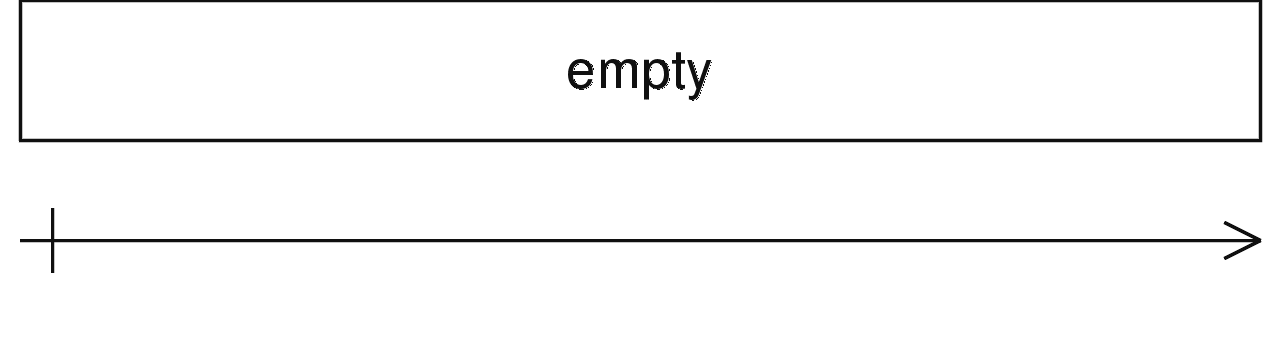/RxJS

# empty

`function` `deprecated`

Creates an Observable that emits no items to the Observer and immediately emits a complete notification.

## Deprecation Notes

Deprecated in favor of using EMPTY constant, or `scheduled` (e.g. `scheduled([], scheduler)`)

### `empty(scheduler?: SchedulerLike)`

#### Parameters

 scheduler Optional. Default is `undefined`. A `SchedulerLike` to use for scheduling the emission of the complete notification.

## Description

Just emits 'complete', and nothing else.This static operator is useful for creating a simple Observable that only emits the complete notification. It can be used for composing with other Observables, such as in a `mergeMap`.

## Examples

#### Emit the number 7, then complete

```import { empty } from 'rxjs';
import { startWith } from 'rxjs/operators';

const result = empty().pipe(startWith(7));
result.subscribe(x => console.log(x));```

#### Map and flatten only odd numbers to the sequence 'a', 'b', 'c'

```import { empty, interval, of } from 'rxjs';
import { mergeMap } from 'rxjs/operators';

const interval\$ = interval(1000);
const result = interval\$.pipe(
mergeMap(x => x % 2 === 1 ? of('a', 'b', 'c') : empty()),
);
result.subscribe(x => console.log(x));

// Results in the following to the console:
// x is equal to the count on the interval eg(0,1,2,3,...)
// x will occur every 1000ms
// if x % 2 is equal to 1 print abc
// if x % 2 is not equal to 1 nothing will be output```